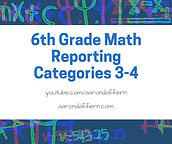#### 6.4h; 6.8a

convert units within a measurement system, including the use of proportions and unit rates; extend previous knowledge of triangles and their properties to include the sum of angles of a triangle, the relationship between the lengths of sides and measures of angles in a triangle, and determining when three lengths form a triangle

#### 6.12c; 6.12d

summarize numeric data with numerical summaries, including the mean and median (measures of center) and the range and interquartile range (IQR) (measures of spread), and use these summaries to describe the center, spread, and shape of the data distribution; summarize categorical data with numerical and graphical summaries, including the mode, the percent of values in each category (relative frequency table), and the percent bar graph, and use these summaries to describe the data distribution

#### 6.14g; 6.14h

explain various methods to pay for college, including through savings, grants, scholarships, student loans, and work-study; compare the annual salary of several occupations requiring various levels of postsecondary education or vocational training and calculate the effects of the different annual salaries on lifetime income

#### 6.8b; 6.8c

model area formulas for parallelograms, trapezoids, and triangles by decomposing and rearranging parts of these shapes; write equations that represent problems related to the area of rectangles, parallelograms, trapezoids, and triangles and volume of right rectangular prisms where dimensions are positive rational numbers

#### 6.13a; 6.14a

interpret numeric data summarized in dot plots, stem-and-leaf plots, histograms, and box plots; compare the features and costs of a checking account and a debit card offered by different local financial institutions

#### 6.8d; 6.11a

determine solutions for problems involving the area of rectangles, parallelograms, trapezoids, and triangles and volume of right rectangular prisms where dimensions are positive rational numbers; graph points in all four quadrants using ordered pairs of rational numbers

#### 6.14b; 6.14c

distinguish between debit cards and credit cards; balance a check register that includes deposits, withdrawals, and transfers

#### 6.12a; 6.12b

represent numeric data graphically, including dot plots, stem-and-leaf plots, histograms, and box plots; use the graphical representation of numeric data to describe the center, spread, and shape of the data distribution

#### 6.14e; 6.14f

describe the information in a credit report and how long it is retained; describe the value of credit reports to borrowers and to lenders

## Quizzes

Click the button below to access the quizzes for each part. Credit will not be earned until the CPE course has been purchased and all quizzes have been completed.

Take the 12 hour CPE course (\$40)

## 6th Grade Math Reporting Categories 3-4